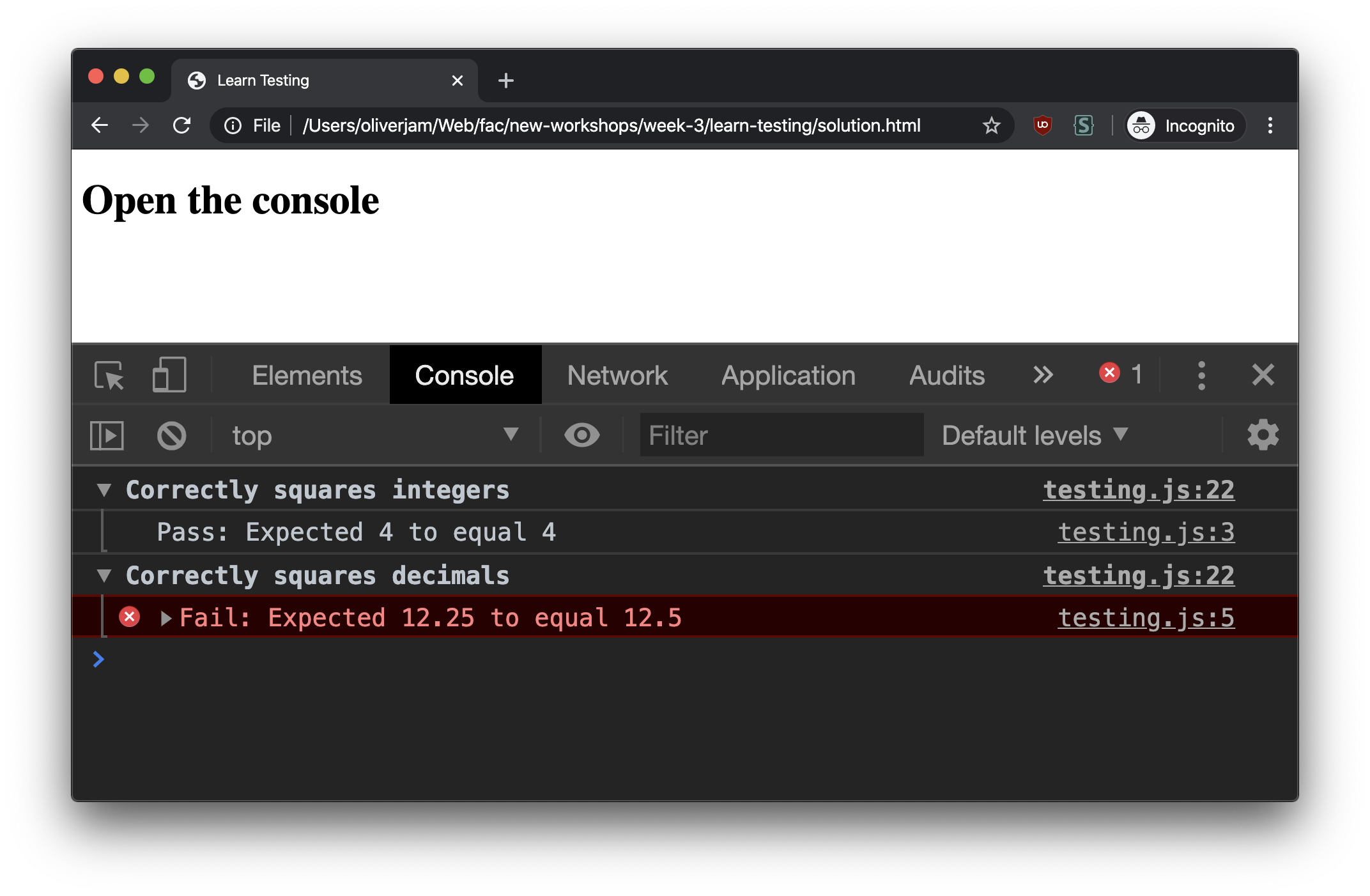# Learn testing in JavaScript

• js
• testing
• fundamentals

The concept of testing code is often introduced with complex libraries. This hides the core of testing: writing some code that runs your other code and tells you if it’s wrong. This workshop introduces the concept by slowly building up a useful function that helps you test your code.

## Setup

2. Open `starter-files/workshop.html` in your editor

There should be a function that squares a number (multiplies it by itself). It’s used like this:

``const result = square(2);console.log(result); // 4``

Right now we only have one option for checking this works. We have to call the function, log the result, then check that result (with a calculator for bigger numbers).

If you want to check it works for lots of different numbers you’ll be doing a bunch of manual work. You’ll have to repeat this work every time the code changes (to make sure you didn’t break it). It would be helpful to automate this process.

## Begin to automate

Since you know how to code you can begin to automate this! Write some JavaScript that calls the `square` function (like above), then checks that the result is what you expect. It should log a useful message to the console using `console.error("my message")` if the result is wrong.

``const result = square(2);const expected = 999; // deliberate wrong answer so we can see a test failureif (result !== expected) {  console.error("Failed: expected square(2) to equal 4, but got " + result);}``

If your test passes change your expected value so that it’s definitely wrong. Can you see the failure in your browser console?

## Make it reusable

This is better than manually checking, but not much. We have to write all the same logic for checking whether the values are the same and logging every time. It would be a lot of copy/pasting to write 20 tests.

### `equal`

Most tests check whether two things are equal. It’s helpful if we extract that logic into a reusable function.

Write a function called `equal` that takes two arguments and checks if they’re the same. If they are it should log the success with `console.info`. If they aren’t it should log the failure with `console.error`.

Use this `equal` function to refactor your test above, then write another one to check that `square(3.5)` is correct.

``function equal(actual, expected) {  if (actual === expected) {    console.info(`Pass: Expected \${expected} and received \${actual}`);  } else {    console.error(`Fail: Expected \${expected} but received \${actual} instead`);  }}const result1 = square(2);const expected1 = 4;equal(result1, expected1);const result2 = square(3.5);const expected2 = 9999; // deliberately wrong answer to cause test to failequal(result2, expected2);``

The first test logs: `"Pass: Expected 4 and received 4`. The second test logs: `Fail: Expected 9999 but received 12.25 instead"` (because we deliberately used the wrong expected value so we could see a test failure).

If your test is passing change your expected value so that it’s definitely wrong. Can you see the error in your browser console?

### `notequal`

It’s sometimes useful to be able to check whether two things are not equal

Write a `notEqual` function. It should be similar to `equal`, but log a failure when its two arguments are the same.

Write a test that checks `square(3)` does not return 10.

``function notEqual(actual, expected) {  if (actual !== expected) {    console.info(`Pass: \${expected} is different to \${actual}`);  } else {    console.error(`Fail: \${expected} is the same as \${actual}`);  }}const result3 = square(3);const expected3 = 10;notEqual(result3, expected3);``

This test logs: `"Pass: 10 is different to 3"`.

## Separating tests

Right now our tests are all jumbled together. This means they share the same scope, so we can’t reuse variable names. It’s also hard to distinguish them in the console. It would be nice if each test had a descriptive name.

We could divide our tests up using functions, like this:

``test("Correctly squares integers", () => {  const result = square(2);  const expected = 4;  equal(result, expected);});test("Correctly squares integers", () => {  const result = square(3.5);  const expected = 12.25;  equal(result, expected);});``

We call a `test` function with a descriptive name, and pass a callback function containing our test code.

Write a function called `test` that takes two arguments: a `name` and a `testFunction`. It should use `console.group` to log a group labelled with the `name`. You’ll need `console.groupEnd` to close the group at the end.

It should call the `testFunction` callback argument so that the actual test is run.

``function test(name, testFunction) {  console.group(name);  testFunction();  console.groupEnd();}test("Correctly squares integers", () => {  const result = square(2);  const expected = 4;  equal(result, expected);});test("Correctly squares decimals", () => {  const result = square(3.5);  const expected = 12.25;  equal(result, expected);});``

See how we can reuse the `result` and `expect` variable names? Each test is self-contained within its own function.## Custom messages

For more complex assertions it’s nice to be able to write a custom message specific to that test.

Amend your `equal` and `notEqual` functions so that they take a third optional `message` argument. Your `console.info`/`console.error` should log this message. If there is no `message` passed in then default to the message you were using before.

``function equal(actual, expected, message) {  if (actual === expected) {    const defaultMessage = `Expected \${expected} and received \${actual}`;    console.info("Pass: " + (message || defaultMessage));  } else {    const defaultMessage = `Expected \${expected} but received \${actual} instead`;    console.error("Fail: " + (message || defaultMessage));  }}function notEqual(actual, expected, message) {  if (actual !== expected) {    const defaultMessage = `\${expected} is different to \${actual}`;    console.info("Pass: " + (message || defaultMessage));  } else {    const defaultMessage = `\${expected} is the same as \${actual}`;    console.error("Fail: " + (message || defaultMessage));  }}test("Correctly squares integers", () => {  const result = square(2);  const expected = 4;  equal(result, expected, "square(2) should return 4");});``
This should log our custom message: `"Pass: square(2) should return 4"`.